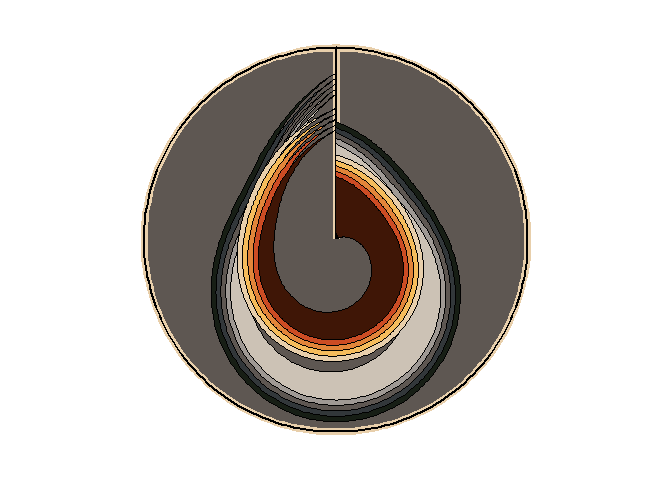MexBrewer is a package with color palettes inspired by the works of Mexican painters and muralists. This package was motivated and draws heavily from the code of Blake R. Mills’s {MetBrewer}, the package with color palettes form the Metropolitan Museum of Art of New York. The structure of the package and coding, like {MetBrewer}, are based on {PNWColors} and {wesanderson}.

## Installation

The package is available from CRAN:

install.packages("MexBrewer")

The development version of the package can be installed like so:

if (!require("remotes")) install.packages("remotes")
remotes::install_github("paezha/MexBrewer") 

## Artists

### Electa Arenal

#### Revolución

This palette is called Revolucion.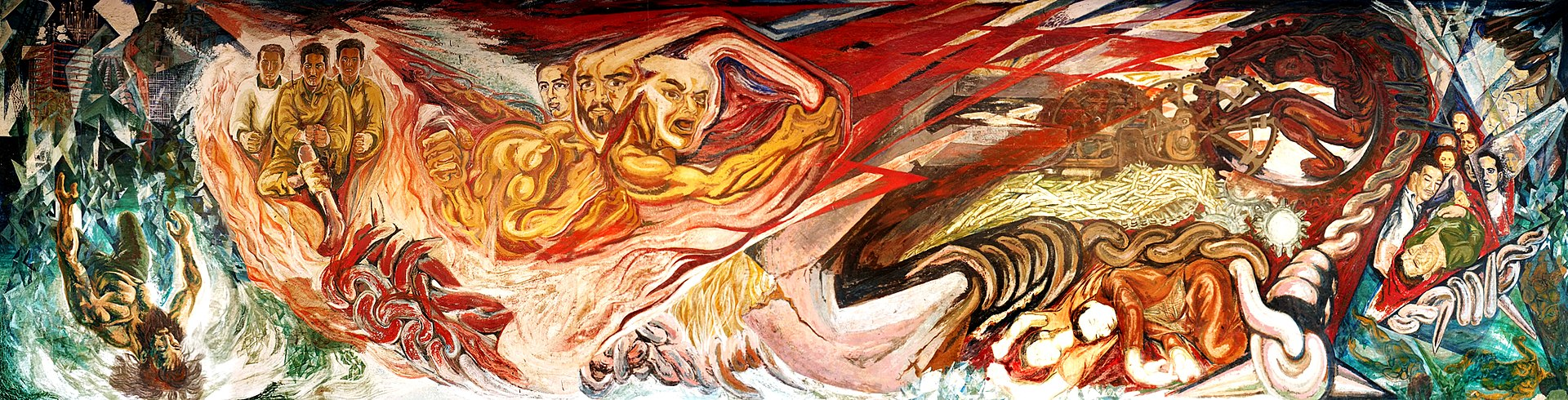Revolucion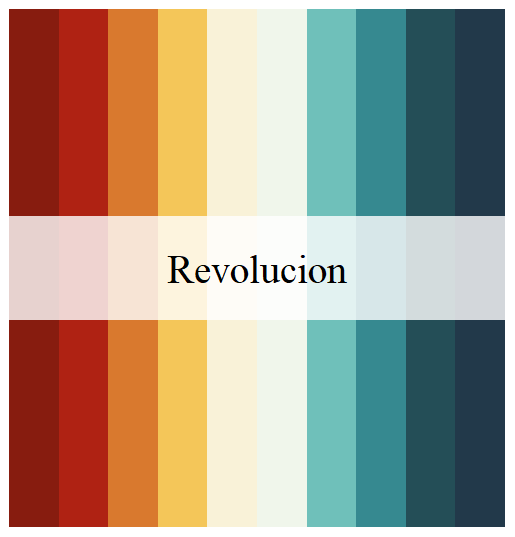Revolucion

### Olga Costa

#### Naturaleza

This palette is called Naturaleza.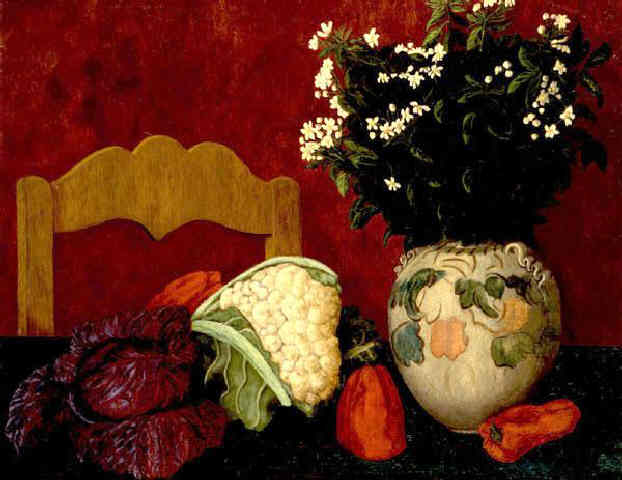Naturaleza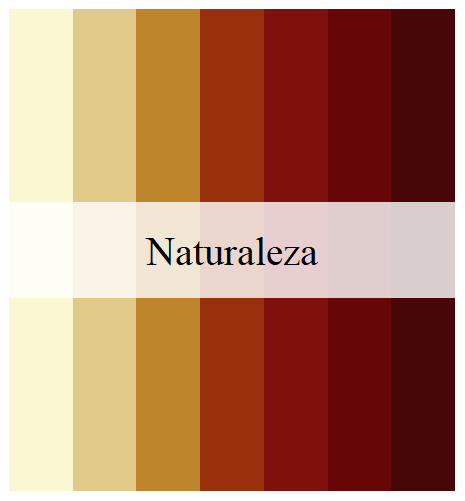Naturaleza

#### Ofrenda

This palette is called Ofrenda.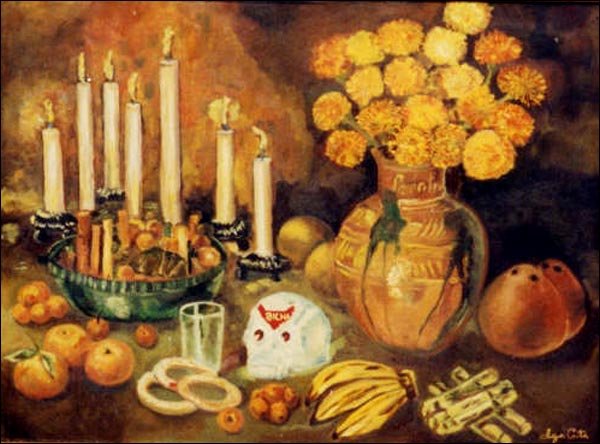Ofrenda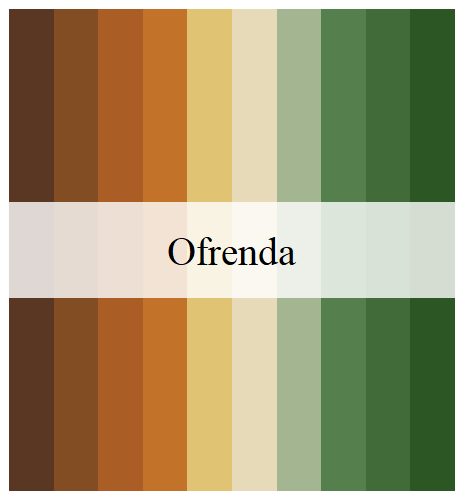Ofrenda

#### Vendedora

This palette is called Vendedora.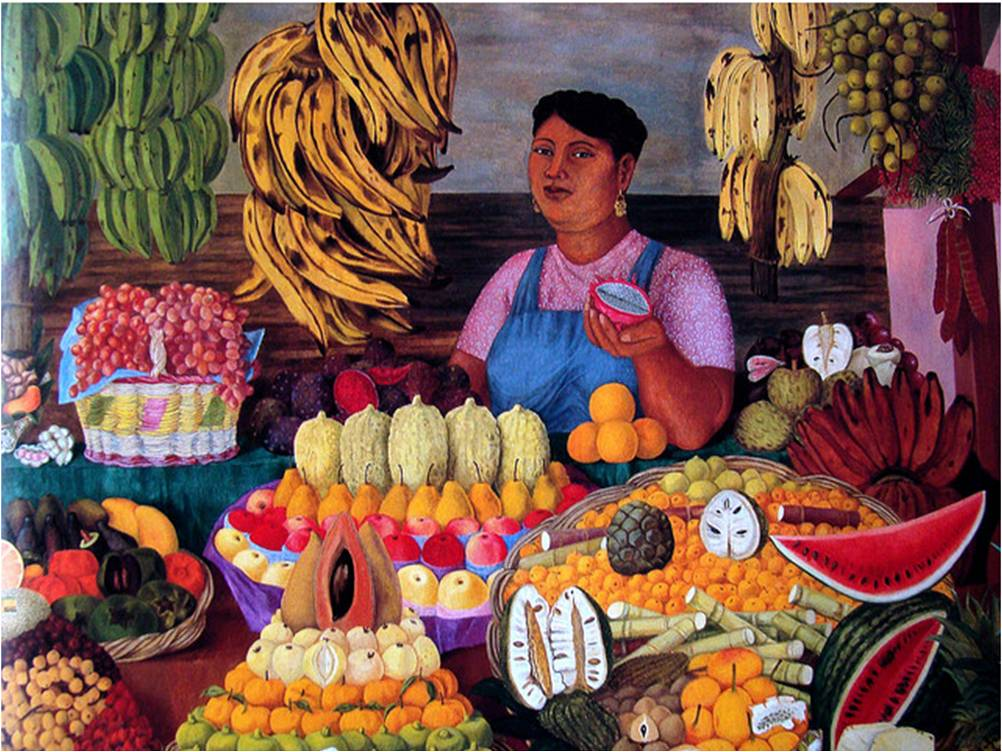Vendedora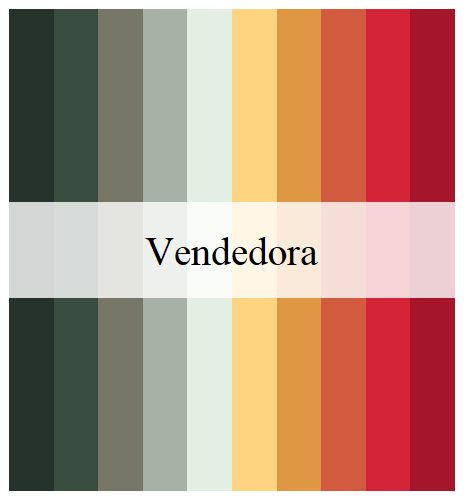Vendedora

### María Izquierdo

#### Alacena

This palette is called Alacena.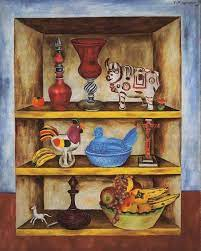Alacena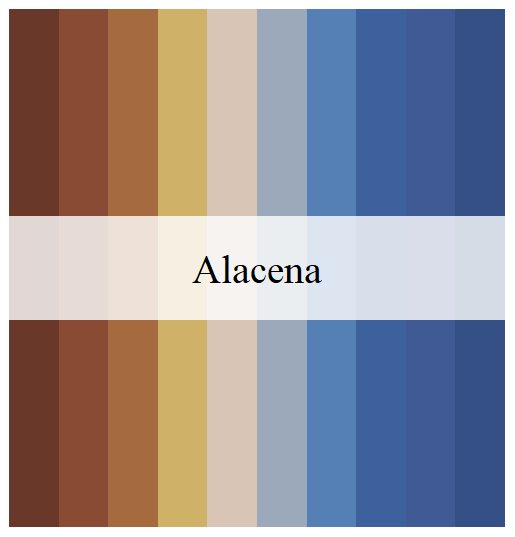Alacena

#### La Tierra

This palette is called Tierra.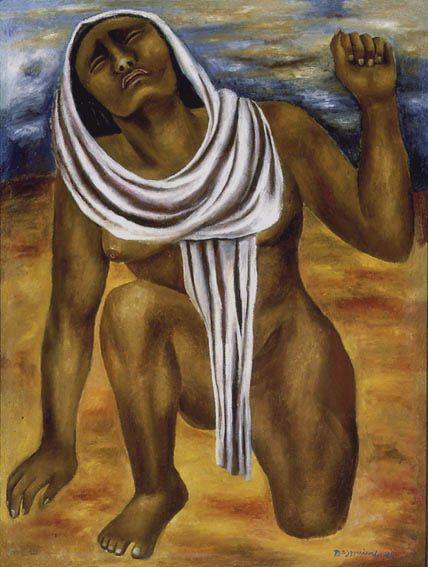Tierra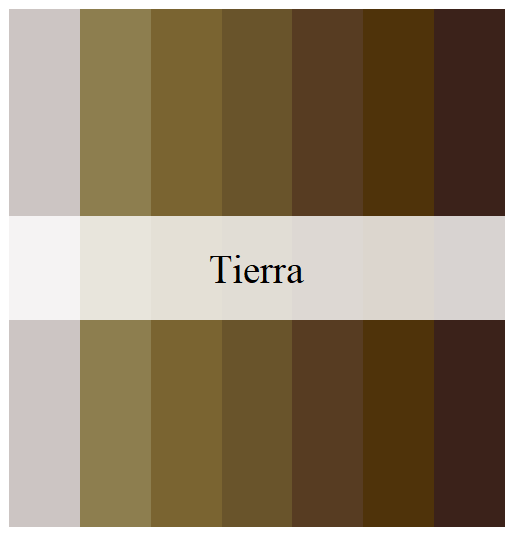Tierra

### Frida Khalo

#### La Casa Azul

These palettes are called Casita1, Casita2, and Casita3. They are inspired by the colors of Frida’s home in Coyoacán, Mexico City.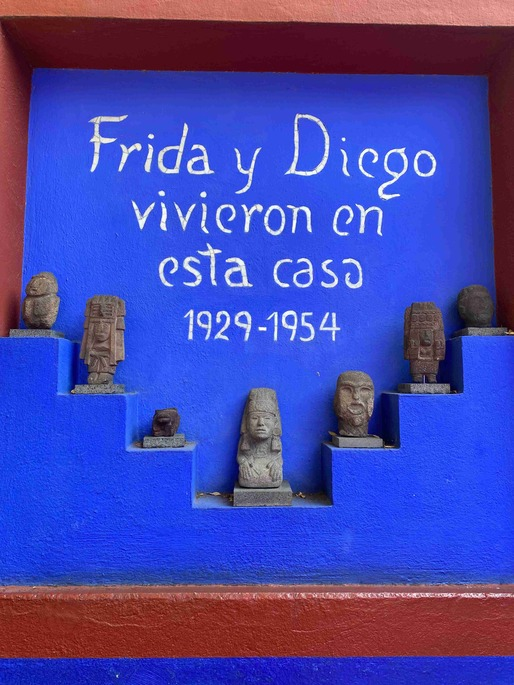Casa Azul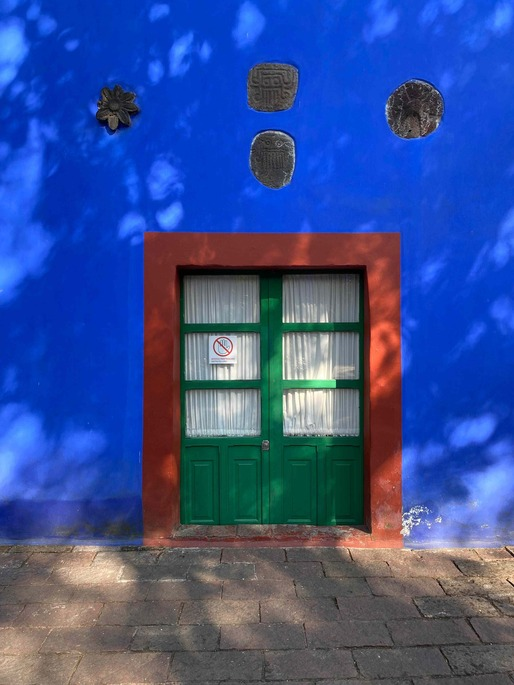Casa Azul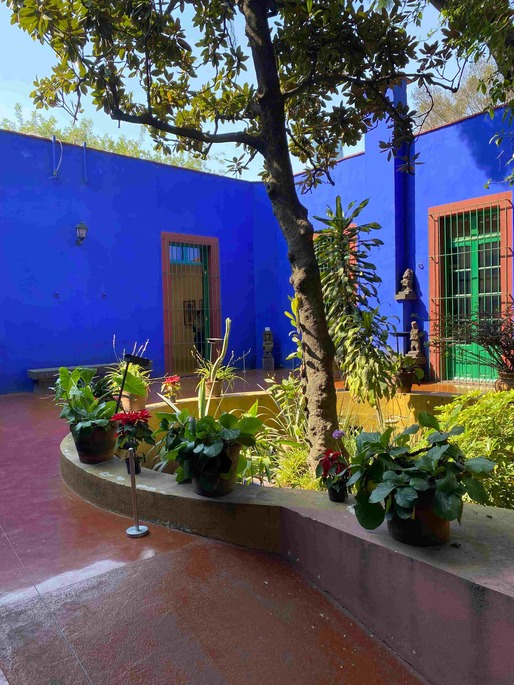Casa Azul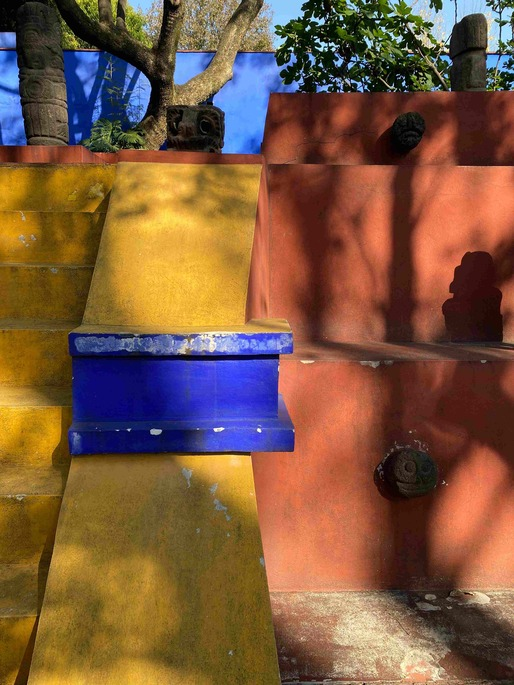Casa Azul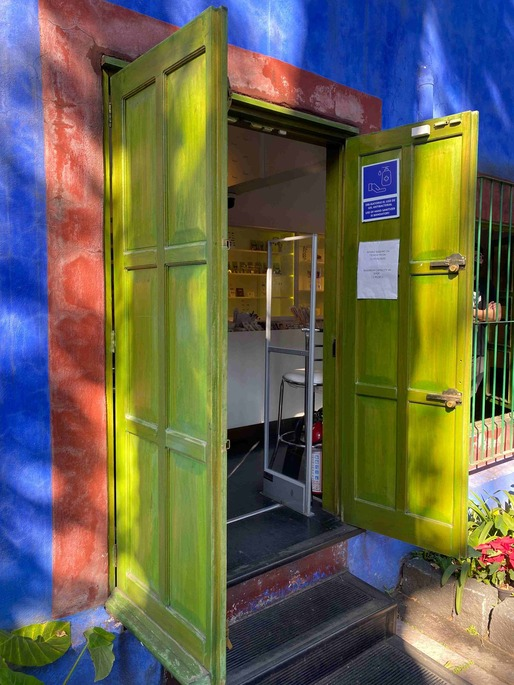Casa Azul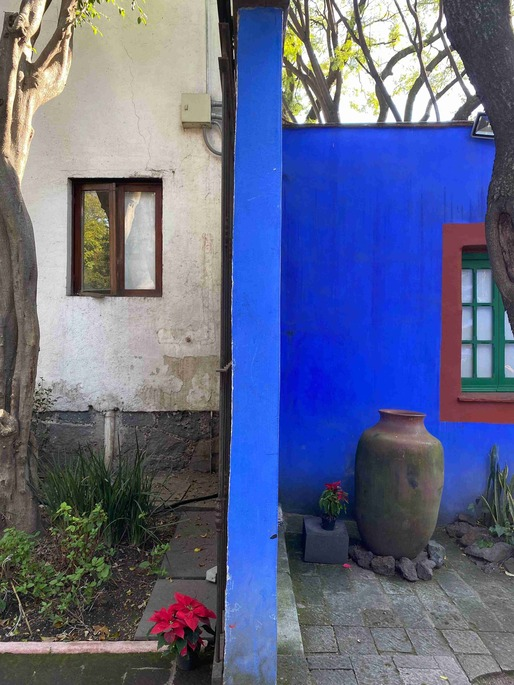Casa Azul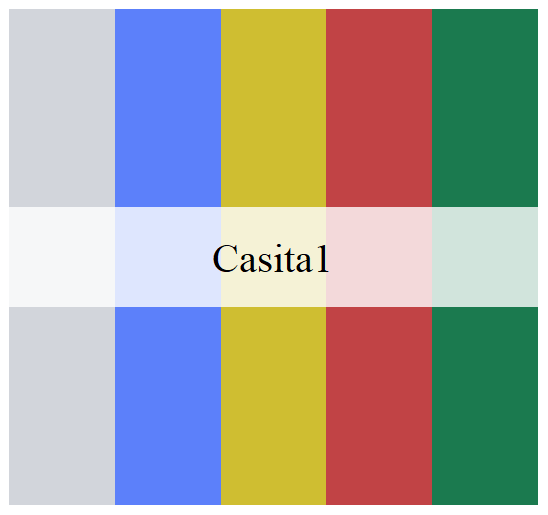Casita1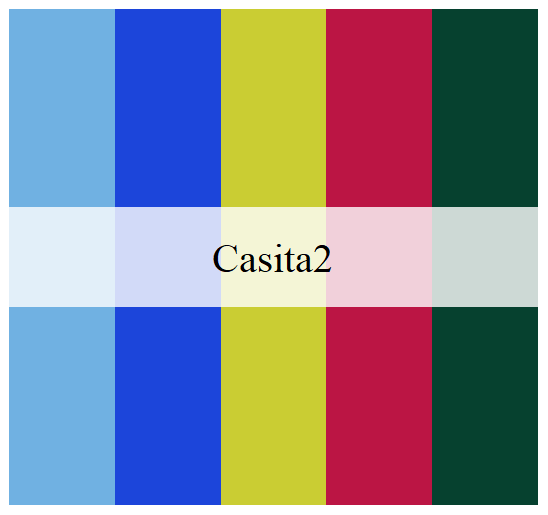Casita2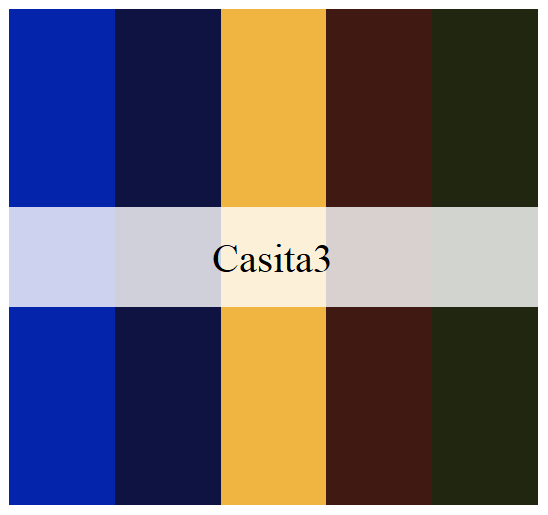Casita3

### Rina Lazo

#### Venerable Abuelo Maiz

This palette is called Maiz.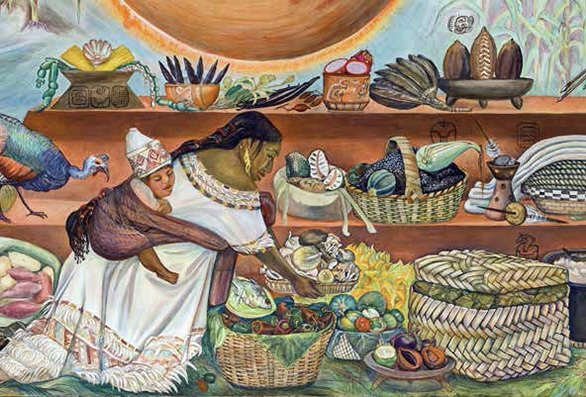Maiz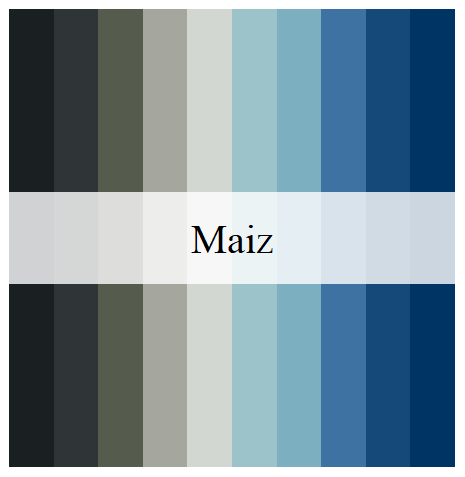Maiz

### Fanny Rabel

#### La Ronda del Tiempo

This palette is called Ronda.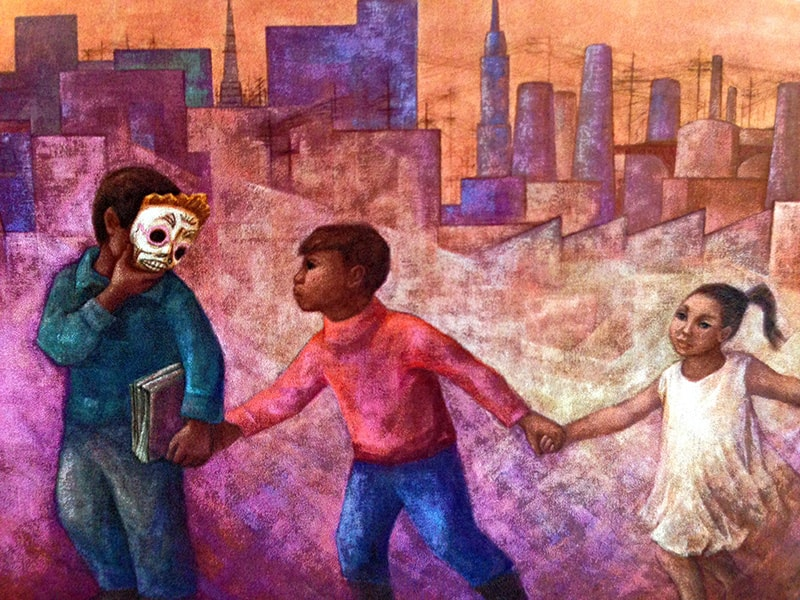Ronda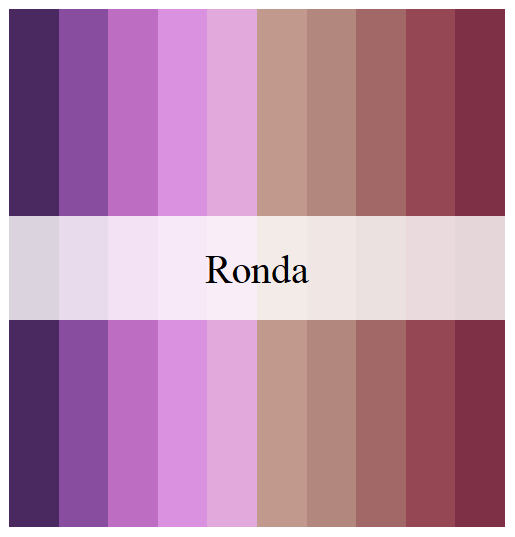Ronda

### Aurora Reyes

#### El atentado a las maestras rurales

This palette is called Atentado.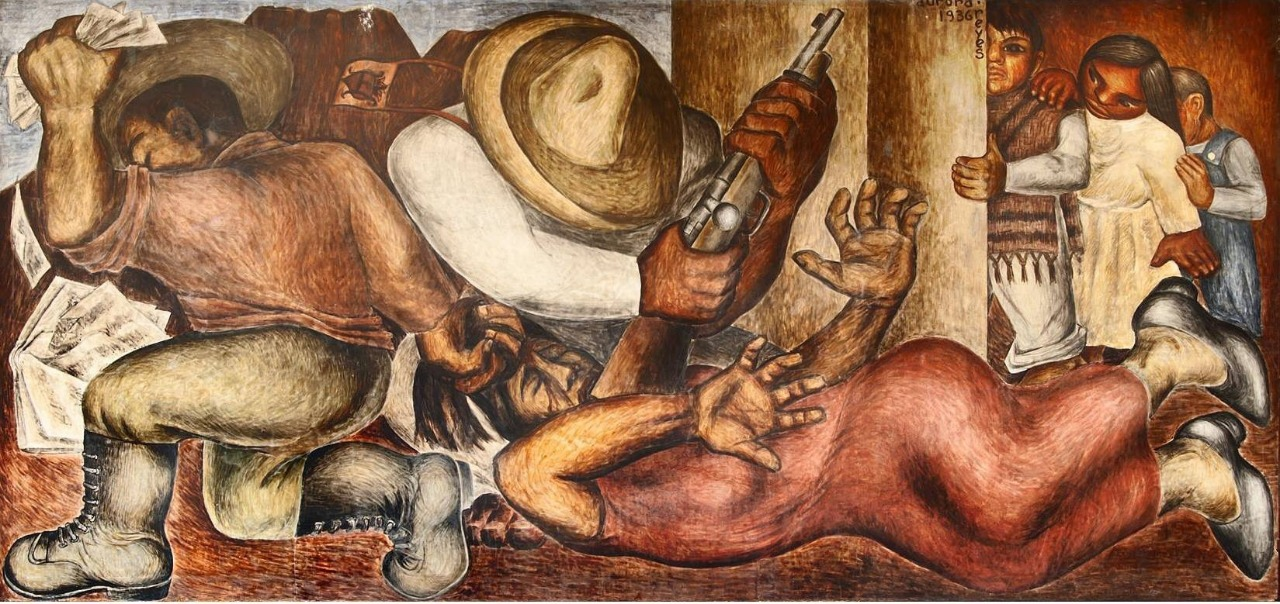Aurora, Concha, y Frida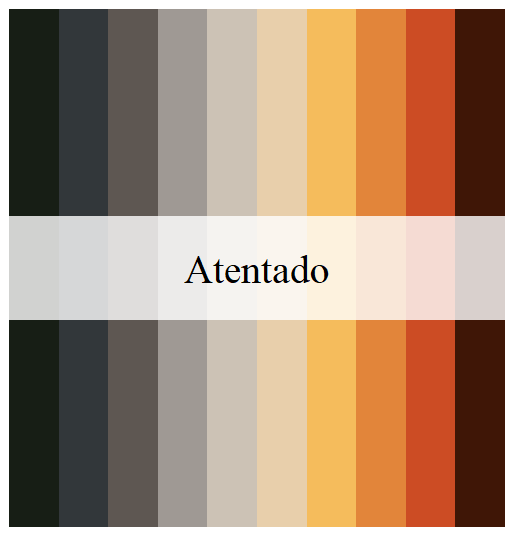Aurora

#### Aurora, Concha, y Frida

This work of Aurora Rivera inspired three palettes, called Aurora, Concha, and Frida.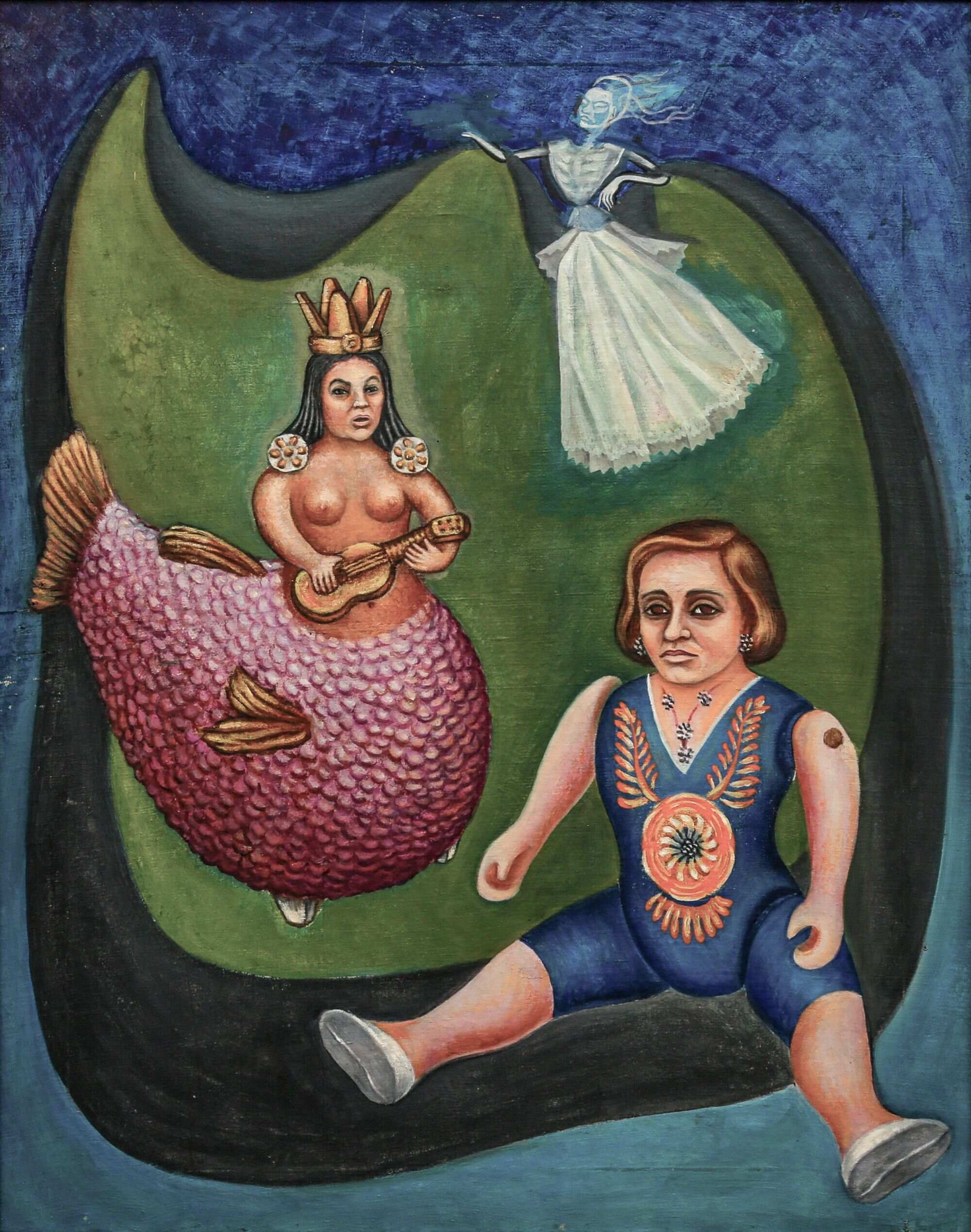Aurora, Concha, y Frida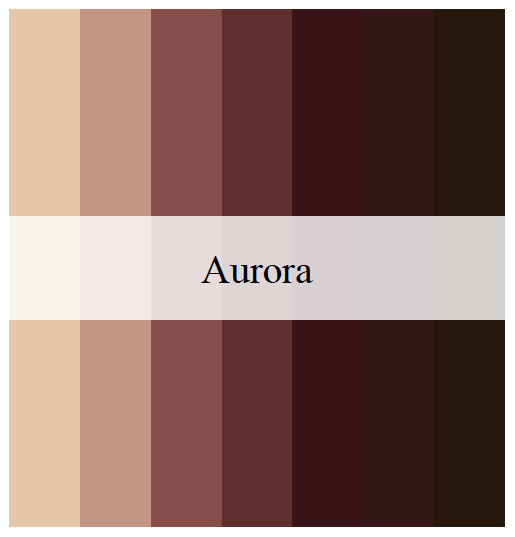Aurora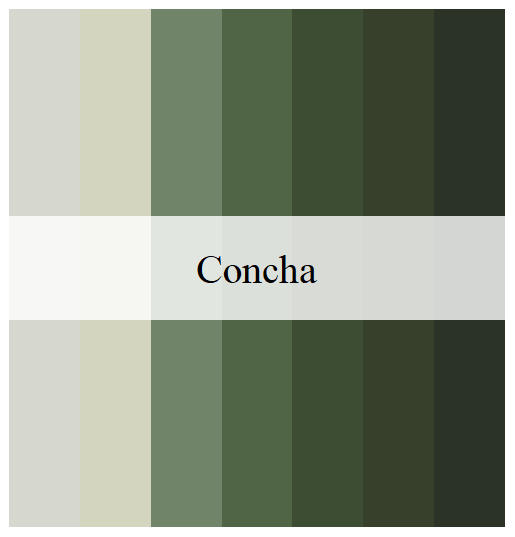Concha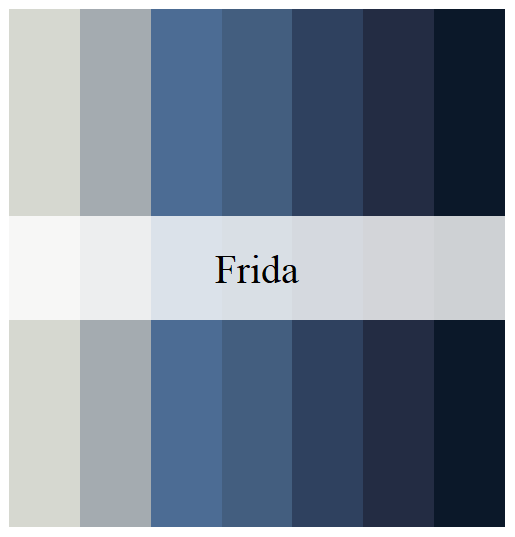Frida

### Remedios Varo

#### La Huida

This palette is called Huida.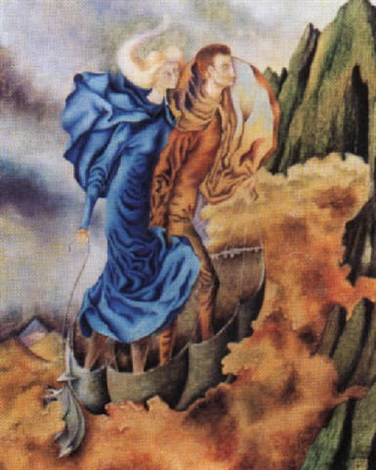La HuidaHuida

#### Taurus

This work of Remedios Varo inspired two palettes, called Taurus1 and Taurus2.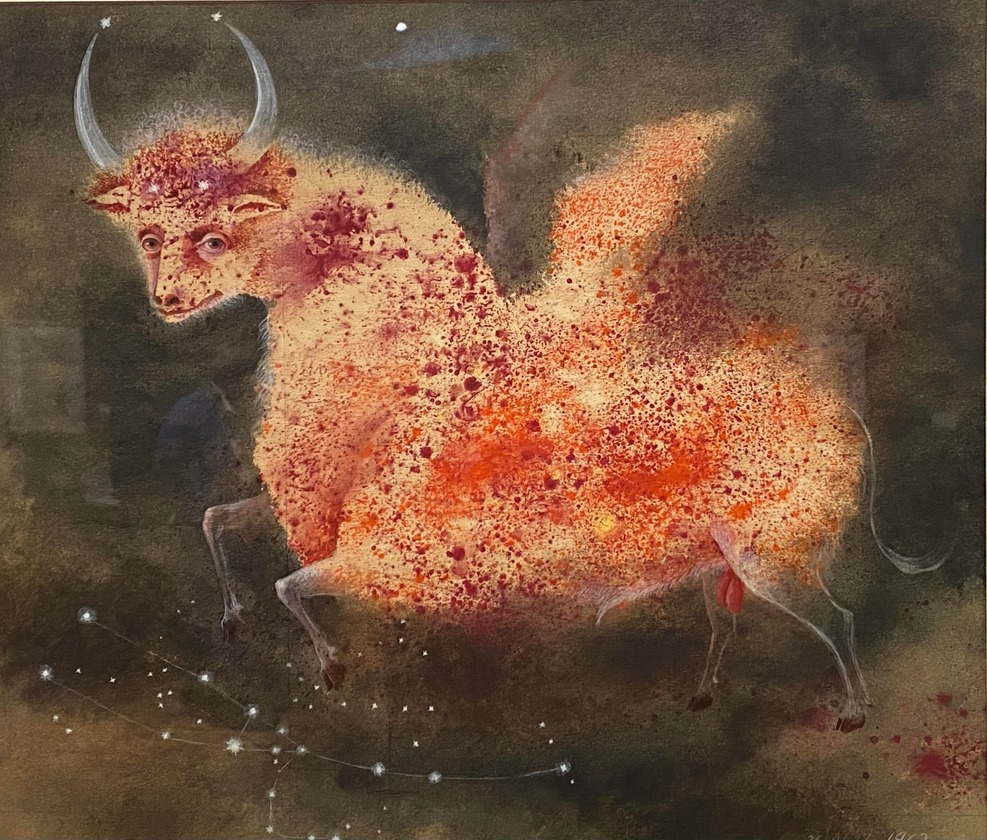Taurus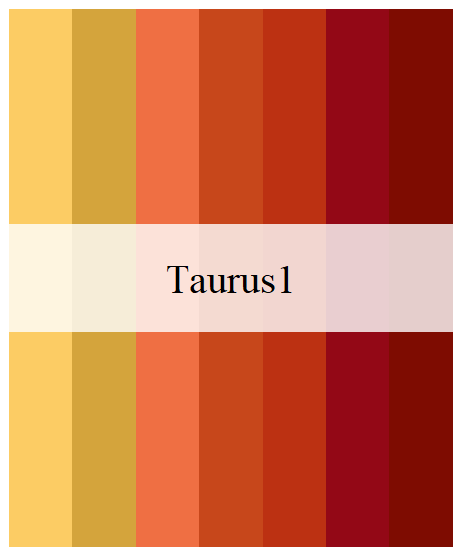Taurus1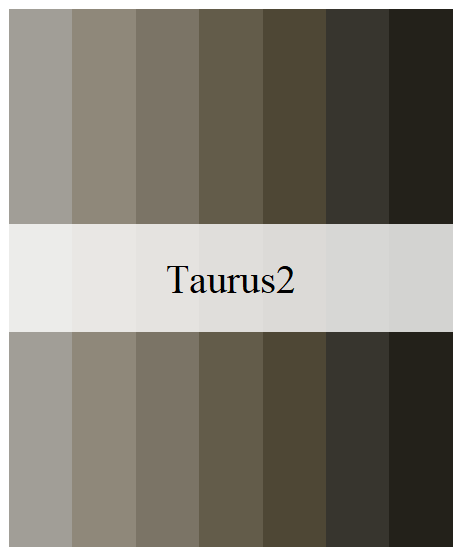Taurus2

## Examples

library(aRtsy) # Koen Derks' package for generative art
library(flametree) # Danielle Navarro's package for generative art
library(MexBrewer)
library(sf)
library(tidyverse)

Invoke data sets used in the examples:

data("mx_estados") # Simple features object with the boundaries of states in Mexico
data("df_mxstate_2020") # Data from {mxmaps }with population statistics at the state level

Join population statistics to state boundaries:

mx_estados <- mx_estados |>
left_join(df_mxstate_2020 |>
#Percentage of population that speak an indigenous language
mutate(pct_ind_lang = indigenous_language/pop * 100) |>
dplyr::transmute(pop2020 = pop,
am2020 = afromexican,
state_name,
pct_ind_lang),
by = c("nombre" = "state_name"))

Distribution of population by geographic region in Mexico:

ggplot(data = mx_estados,
aes(x = region, y = pop2020, fill = region)) +
geom_boxplot() +
scale_fill_manual(values = mex.brewer("Concha", n = 5)) +
theme_minimal()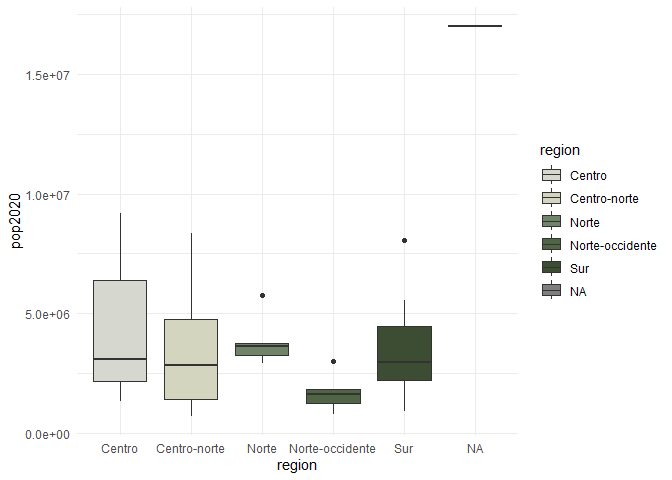Percentage of population who speak an indigenous language in 2020 by state:

ggplot() +
aes(fill = pct_ind_lang),
color = "white",
size = 0.08) +
theme_minimal()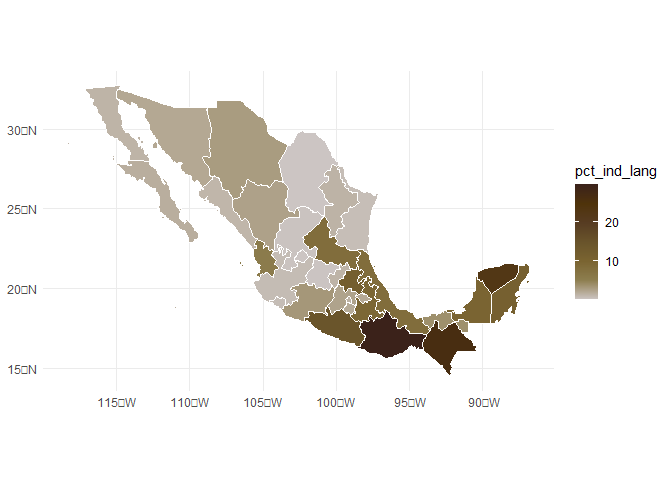# Some Rtistry

### Danielle Navarro’s {flametree}

The following three images were created using the {flametree} package.

# pick some colours
as.vector()

# data structure defining the trees
dat <- flametree_grow(seed = 3563,
time = 11,
trees = 10)

# draw the plot
dat |>
flametree_plot(
style = "nativeflora"
)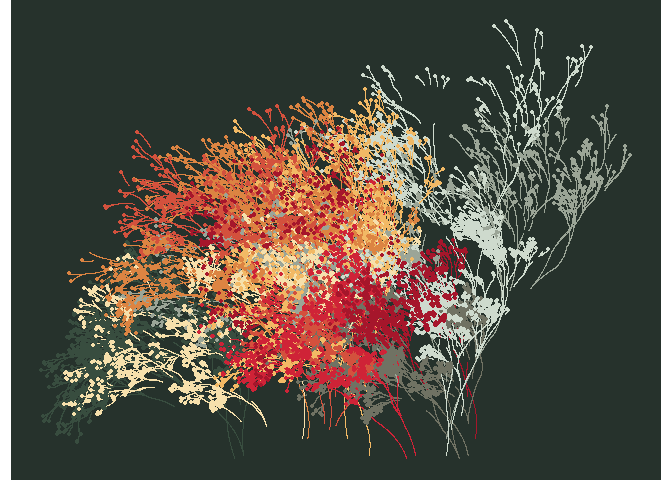# pick some colours
as.vector()

# data structure defining the trees
dat <- flametree_grow(seed = 3536,
time = 8,
trees = 6)

# draw the plot
dat |>
flametree_plot(
style = "wisp"
)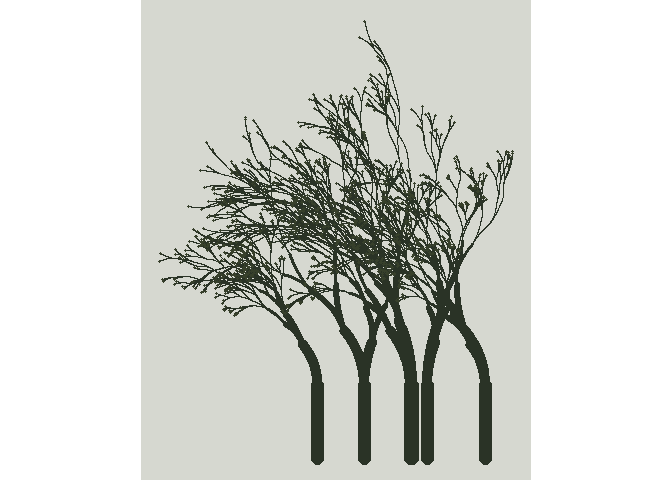# pick some colours
as.vector()

# data structure defining the trees
dat <- flametree_grow(seed = 3653,
time = 8,
trees = 6)

# draw the plot
dat |>
flametree_plot(
style = "minimal"
)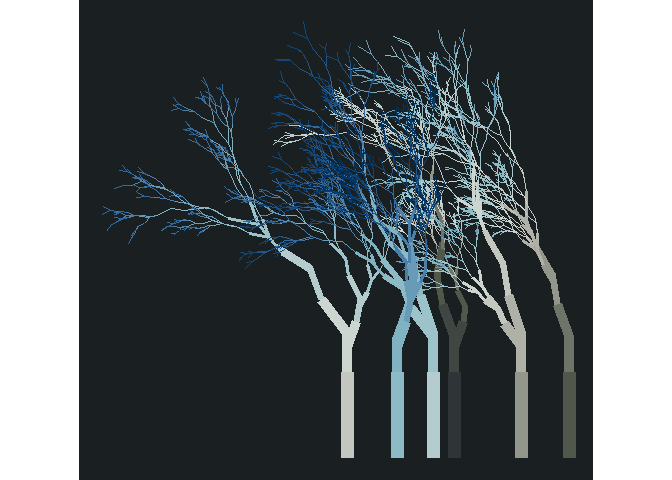### Koen Derks’s aRtsy

The following three images were created using the {aRtsy} package.

Functions:

my_formula <- list(
x = quote(runif(1, -1, 1) * x_i^2 - sin(y_i^2)),
y = quote(runif(1, -1, 1) * y_i^3 - cos(x_i^2))
)

polar = FALSE,
by = 0.005,
formula = my_formula)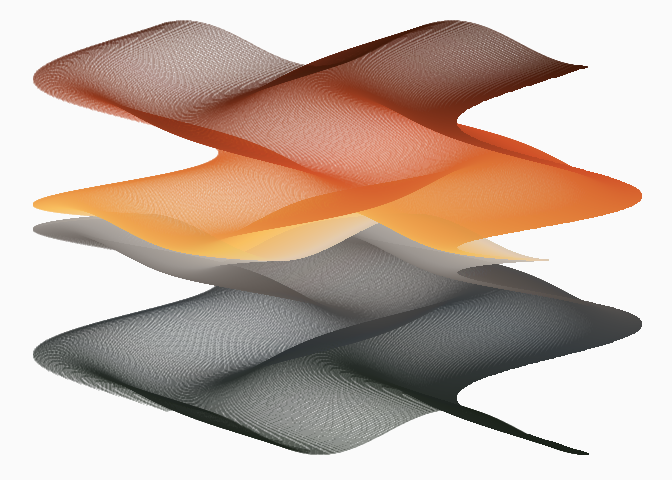Mosaic:

canvas_squares(colors = mex.brewer("Alacena"),
cuts = 20,
ratio = 1.5,
resolution = 200,
noise = TRUE)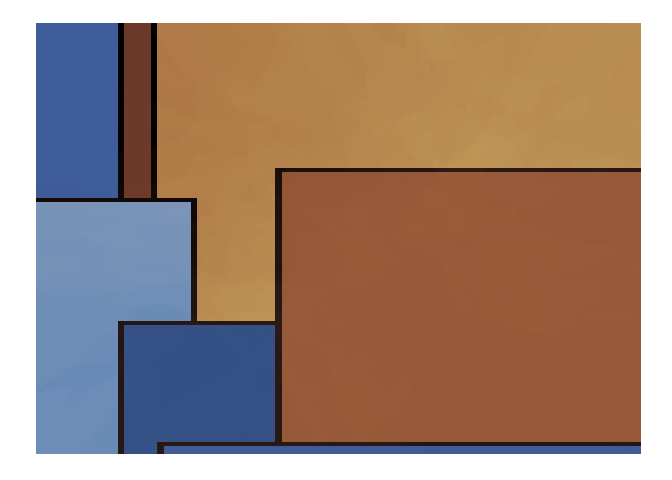Mandelbrot’s set:

canvas_mandelbrot(colors = mex.brewer("Naturaleza"),
zoom = 8,
iterations = 200,
resolution = 500)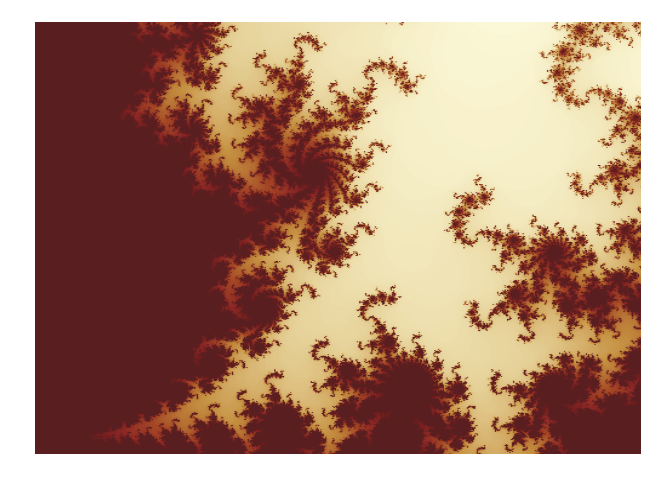### Meghan S. Harris’s waves

These plots are adaptations of Meghan Harris’s artsy waves. Create data frames with wave functions:

##Set up the "range" on the x axis for horizontal waves=====
wave_theta <- seq(from = -pi,
to = -0,
by = 0.01)

# Create waves using functions
wave_1 <- data.frame(x = wave_theta) |>
mutate(y = (sin(x) * cos(2 * wave_theta) + exp(x * 2)))

wave_2 <- data.frame(x = wave_theta) |>
mutate(y = (0.5 * sin(x) * cos(2.0 * wave_theta) + exp(x)) - 0.5)

Define a function to convert a single wave into a set of n waves. The function takes a data frame with a wave function and returns a data frame with n waves:

# Creating a function for iterations====

wave_maker <- function(wave_df, n, shift){

#Create an empty list to store our multiple dataframes(waves)#
wave_list<- list()

#Create a for loop to iteratively make "n" waves shifted a distance shift from each other #
for(i in seq_along(1:n)){

wave_list[[i]] <- wave_df |>
mutate(y = y - (shift * i),
group = i)
}

#return the completed data frame to the environment#
return(bind_rows(wave_list))
}

Create layered waves using the data frames with the wave functions above:

wave_layers <- rbind(wave_1 |>
wave_maker(n = 5,
shift = 0.075),
wave_2 |>
wave_maker(n = 5,
shift = 0.075) |>
mutate(group = group + 5)) # adjust the group counter to identify waves uniquely

Plot layered waves using cartesian coordinates and palette Ofrenda:

ggplot(wave_layers) +
geom_rect(aes(xmin = -pi,
xmax = -0.0,
ymin = min(y) - 0.50,
ymax = max(y) + 0.30 ),
size = 2.5,
color = mex.brewer("Ofrenda"),
fill = mex.brewer("Ofrenda")) +
geom_rect(aes(xmin = -pi,
xmax = -0.0,
ymin = min(y) - 0.50,
ymax = max(y) + 0.30 ),
size = 1,
color = "black",
fill = NA) +
geom_ribbon(aes(x,
ymin = y - 0.025 * 4 * x,
ymax = y + 0.015 * 10 * x,
group = group,
fill = group),
color = "black",
size = 0.5) +
theme_void() +
theme(legend.position = "none")
#> Warning: Using size aesthetic for lines was deprecated in ggplot2 3.4.0.
#> ℹ Please use linewidth instead.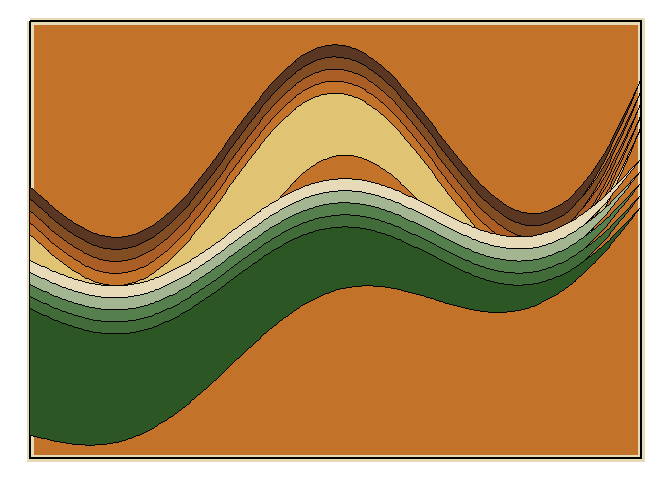Plot layered waves using polar coordinates and palette Atentado:

ggplot(wave_layers) +
geom_rect(aes(xmin = -pi,
xmax = -0.0,
ymin = min(y) - 0.45,
ymax = max(y) + 0.30 ),
size = 2.5,
geom_rect(aes(xmin = -pi,
xmax = -0.0,
ymin = min(y) - 0.45,
ymax = max(y) + 0.30 ),
size = 1,
color = "black",
fill = NA) +
geom_ribbon(aes(x,
ymin = y - 0.025 * 4 * x,
ymax = y + 0.015 * 10 * x,
group = group,
fill = group),
color = "black",
size = 0.5) +
theme(legend.position = "none")